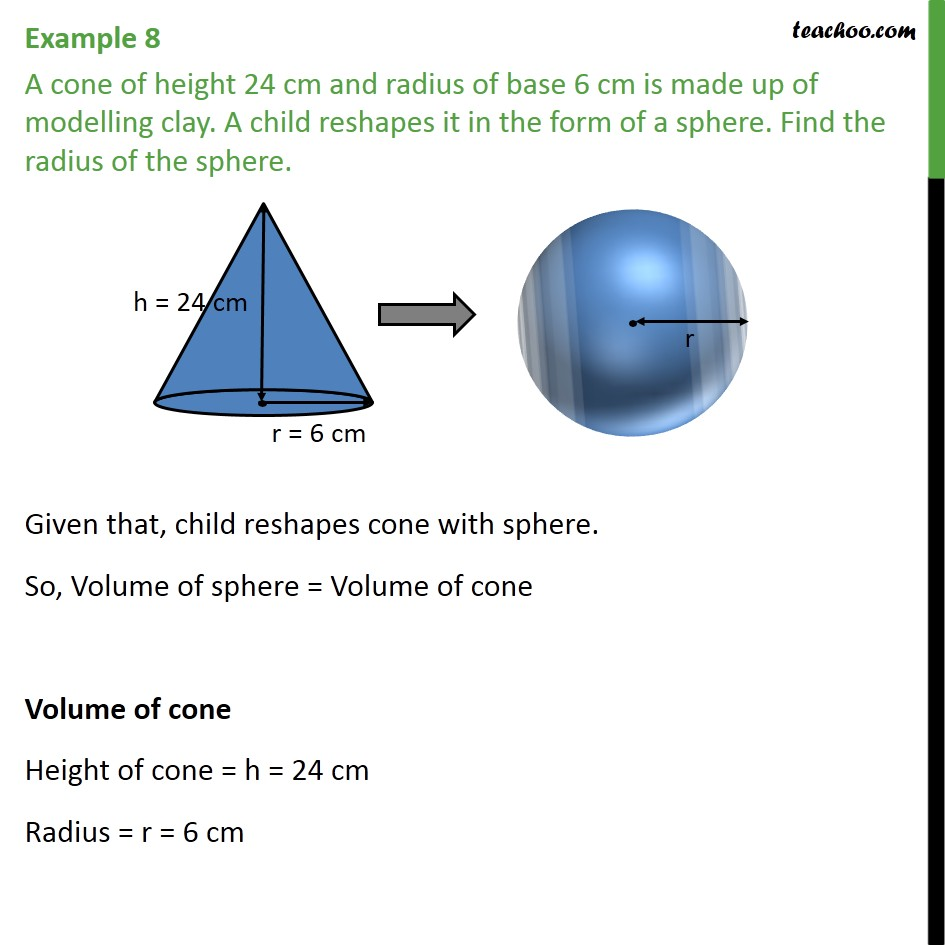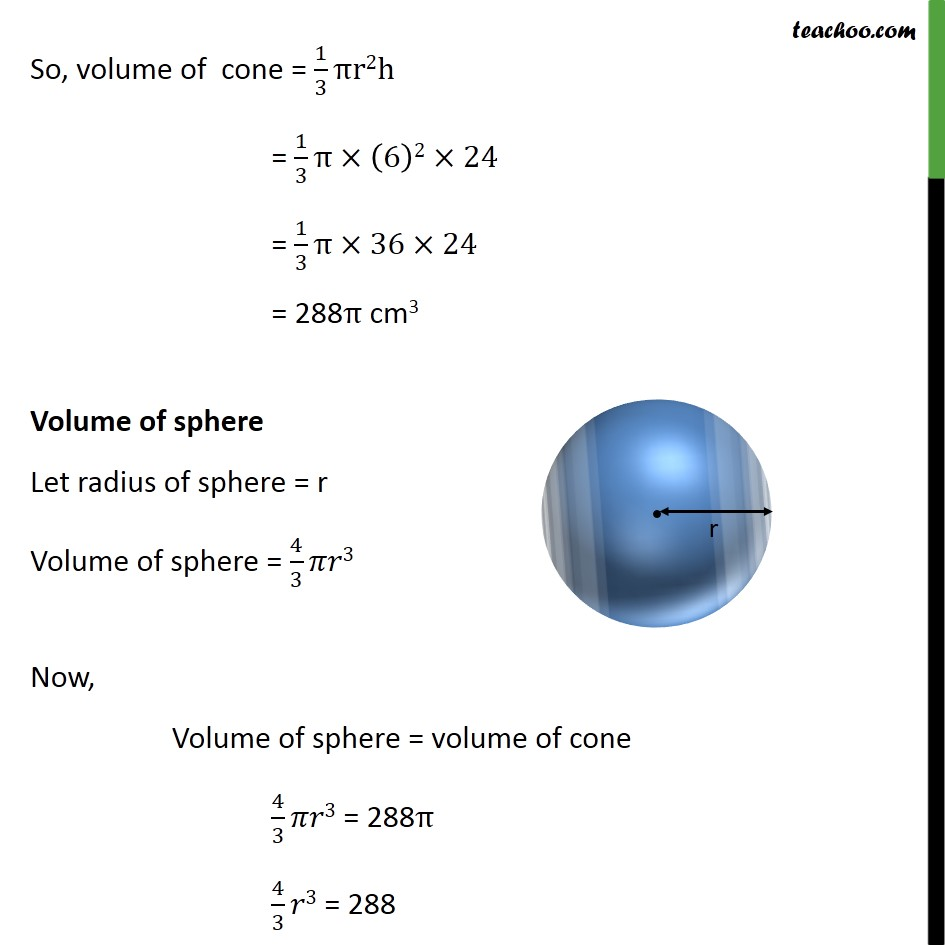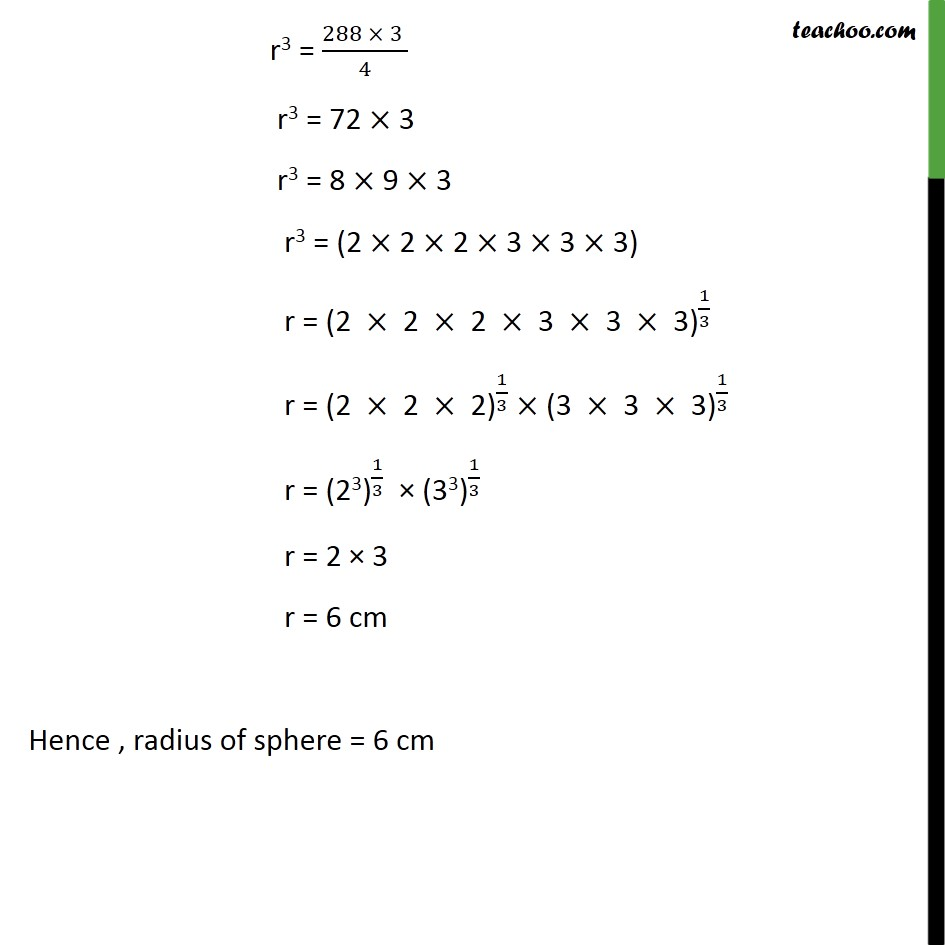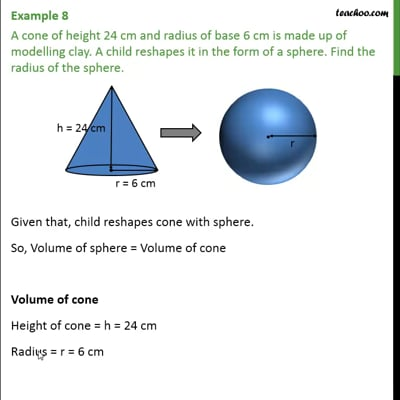Examples

Chapter 13 Class 10 Surface Areas and Volumes
Serial order wiseThis video is only available for Teachoo black users

Solve all your doubts with Teachoo Black (new monthly pack available now!)

### Transcript

Example 8 A cone of height 24 cm and radius of base 6 cm is made up of modelling clay. A child reshapes it in the form of a sphere. Find the radius of the sphere. Given that, child reshapes cone with sphere. So, Volume of sphere = Volume of cone Volume of cone Height of cone = h = 24 cm Radius = r = 6 cm So, volume of cone = 1/3 πr2h = 1/3 π×(6)2×24 = 1/3 π×36×24 = 288π cm3 Volume of sphere Let radius of sphere = r Volume of sphere = 4/3 𝜋𝑟3 Now, Volume of sphere = volume of cone 4/3 𝜋𝑟3 = 288π 4/3 𝑟3 = 288 r3 = (288 × 3 )/4 r3 = 72 × 3 r3 = 8 × 9 × 3 r3 = (2 × 2 × 2 × 3 × 3 × 3) r = 〖"(2 " ×" 2 " ×" 2 " ×" 3 " ×" 3 " ×" 3)" 〗^(1/3) r = 〖"(2 " ×" 2 " ×" 2)" 〗^(1/3) × 〖"(3 " ×" 3 " ×" 3)" 〗^(1/3) r = 〖"(23)" 〗^(1/3) × 〖"(33)" 〗^(1/3) r = 2 × 3 r = 6 cm Hence , radius of sphere = 6 cm